﻿ 基于EDEM的行星式混凝土搅拌机关键结构优化设计

# 基于EDEM的行星式混凝土搅拌机关键结构优化设计Key Structure Optimization Design of Planetary Concrete Mixer Based on EDEM

Abstract: Planetary concrete mixers have been widely used due to their high mixing efficiency, and their structure directly affects the mixing quality and efficiency of concrete. In order to optimize its structure, the stirring efficiency is improved. Taking the structure of the planetary concrete mixer produced by a company as a reference, the discrete element software EDEM was used to simulate material agitation, and the flow state of the material and the uniformity of material mixing were observed. It is found that the material movement at the sidewall of the mixing tank during the mixing process is single, and local agitation is uneven. For this phenomenon, after the shape of the scraper arm of the mixer is changed from plane to circular surface, the simulation process is re-peated. It shows that the overall mixing uniformity of the mixer after structural optimization has a significant improvement.

1. 引言

2. 行星式搅拌机结构分析1.驱动电机 2.机架 3.行星齿轮系 4.搅拌臂 5.铲板 6.边刮臂

Figure 1. 3D model of planetary mixer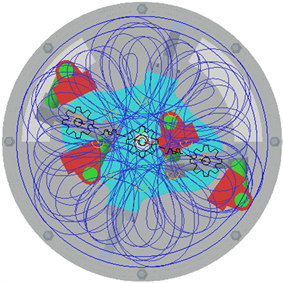Figure 2. Trajectory of shovel plate

3. EDEM仿真分析

3.1. EDEM简介及模型的建立

3.2. 仿真参数的设置

$U=-\text{π}{a}^{2}\gamma$ (1)

$\gamma ={\gamma }_{1}+{\gamma }_{2}+{\gamma }_{12}$ (2)

${F}_{JKR}=-4\sqrt{\text{π}\gamma {\alpha }^{3}{E}^{*}}+\frac{4{E}^{*}{\alpha }^{3}}{3R}$ (3)

$\delta =\frac{{\alpha }^{2}}{R}-\sqrt{\frac{4\text{π}\gamma \alpha }{{E}^{*}}}$ (4)

$\frac{1}{{E}^{*}}=\frac{\left(1-{V}_{1}^{2}\right)}{{E}_{1}}-\frac{\left(1-{V}_{2}^{2}\right)}{{E}_{2}}$ (5)

$\frac{1}{R}=\frac{1}{{R}_{1}}+\frac{1}{{R}_{2}}$ (6)Table 1. Material property parameter setting

Table 2. Contact parameter setting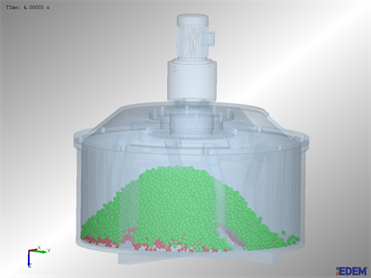Figure 3. Mixture status at the end of feeding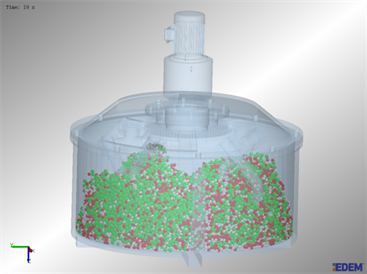Figure 4. Mixture states after 19 s of mixing

3.3. 颗粒混合均匀度分析

${C}_{v}=\frac{\sqrt{\underset{1}{\overset{n}{\sum }}\frac{{\left({\epsilon }_{i}-\stackrel{¯}{\epsilon }\right)}^{2}}{n-1}}}{\stackrel{¯}{\epsilon }}$ (7)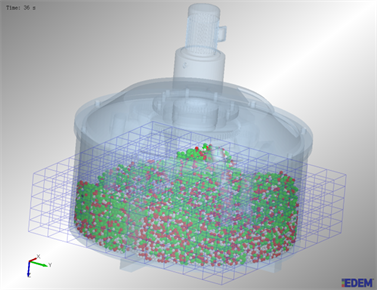Figure 5. Mesh generation of simulation analysis

3.4. 搅拌过程分析

Table 3. Variation of particle mixing uniformity with time before structure optimization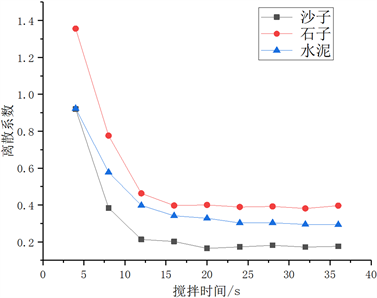Figure 6. Change of particle mixing uniformity during stirring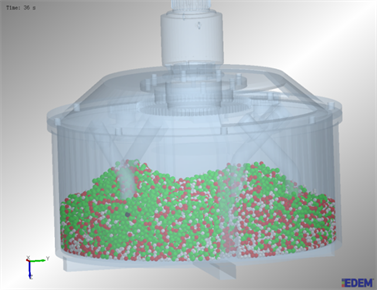Figure 7. Mixing state at the end of mixing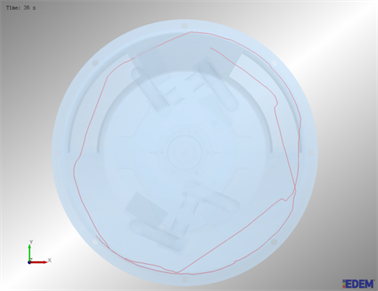Figure 8. Trajectory of labeled particles

4. 结构优化

4.1. 边刮臂的结构优化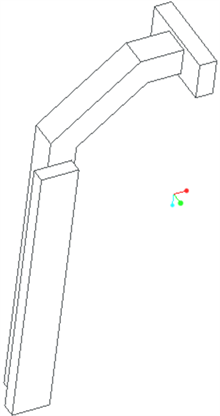Figure 9. Edge scraper arm before structure optimization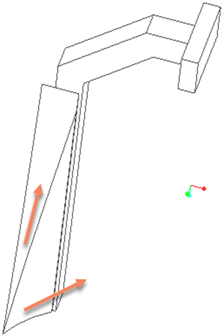Figure 10. Edge scraper arm after structure optimization

4.2. 搅拌结果对比(a) 结构改进后搅拌效果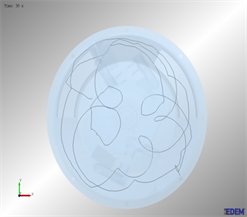(b) 俯视图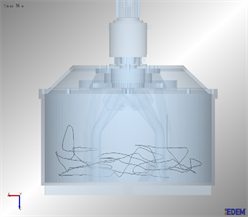(c) 主视图

Figure 11. Trajectory of labeled particles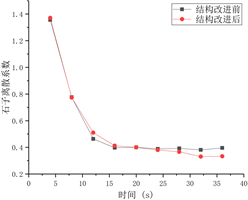(a) 石子离散系数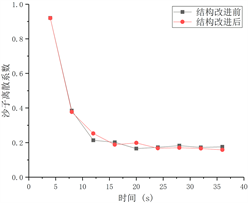(b) 沙子离散系数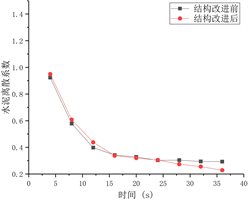(c) 水泥离散系数

Figure 12. Comparison of mixing uniformity results

5. 结论

 王玄丰, 赵悟, 汤钊, 王敬. 基于EDEM的双立轴搅拌机拌缸倾角对比研究[J]. 机械研究与应用, 2018, 31(6): 10-13.

 冯忠绪. 搅拌理论及其设备的研究进展[J]. 工程机械, 2014, 45(5): 1-8.

 张军. 基于EDEM的混凝土搅拌机搅拌过程的料流分析[J]. 筑路机械与施工机械化, 2018, 35(8): 102-106.

 董玉刚, 林亮亮, 孔鲜宁, 刘云飞. 基于EDEM的双卧轴搅拌机搅拌工艺对比研究[J]. 水泥工程, 2016(2): 87-90.

 姚运仕, 刘欢建, 任峰, 张良奇, 孔鲜宁, 李冠峰, 刘其昂, 张梦荻. 高性能混凝土振动搅拌试验研究[J]. 硅酸盐通报, 2020, 39(3): 730-733.

 纪云, 刘送永. 混凝土搅拌机搅拌性能的离散元分析[J]. 施工技术, 2016, 45(12): 144-146+153.

 胡国明. 颗粒系统的离散元素法分析仿真[M]. 武汉: 武汉理工大学出版社, 2010.

 曹波, 李文辉, 王娜, 白小云, 王程伟. 基于JKR模型的湿式滚抛磨块离散元参数标定[J]. 表面技术, 2019, 48(3): 249-256.

Top